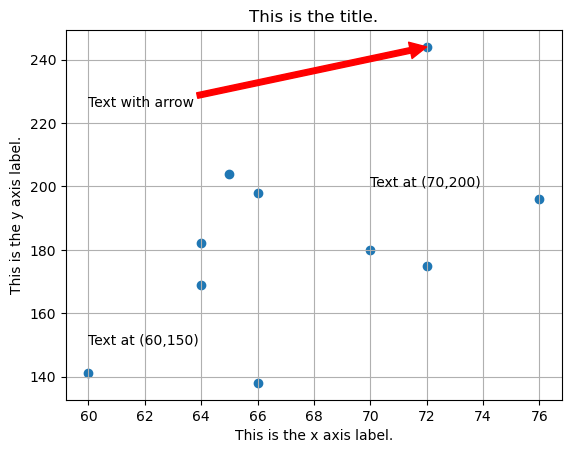# How to add details to a plot

## Description

After making a plot, we might want to add axis labels, a title, gridlines, or text. Plotting packages provide tons of tools for this sort of thing. What are some of the essentials?

Related topics:

## Using Matplotlib, in Python

View this solution alone.

We will create some fake data using Python lists, for simplicity. But everything we show below works also if your data is in columns of a DataFrame, such as df['age'].

1
2
patient_height = [  60,  64,  64,  65,  66,  66,  70,  72,  72,  76 ]
patient_weight = [ 141, 182, 169, 204, 138, 198, 180, 175, 244, 196 ]


The conventional way to import matplotlib in Python is as follows.

1
import matplotlib.pyplot as plt


The following code creates a plot with many details added, but each is independent of the others, so you can take just the bit of code that you need.

1
2
3
4
5
6
7
8
9
10
plt.scatter( patient_height, patient_weight )
plt.xlabel( 'This is the x axis label.' )
plt.ylabel( 'This is the y axis label.' )
plt.title( 'This is the title.' )
plt.grid()                                     # Turns on gridlines
plt.text( 70, 200, 'Text at (70,200)' )        # Text method 1
plt.annotate( 'Text at (60,150)', (60,150) )   # Text method 2
plt.annotate( 'Text with arrow', xytext=(60,225), xy=(72,244),
arrowprops={'color':'red'} )     # Text with arrow
plt.show()See a problem? Tell us or edit the source.

## Solution, in R

View this solution alone.

We will create some fake data using R vectors, for simplicity. But everything we show below works also if your data is in columns of a data frame, such as df\$age.

1
2
patient_height <- c(  60,  64,  64,  65,  66,  66,  70,  72,  72,  76 )
patient_weight <- c( 141, 182, 169, 204, 138, 198, 180, 175, 244, 196 )


The following code creates a plot with many details added, but each is independent of the others, so you can take just the bit of code that you need.

1
2
3
4
5
6
7
8
9
plot( patient_height, patient_weight,
main="This is the title.",
xlab="This is the x axis label.",
ylab="This is the y axis label." )   # Scatter plot with labels
grid()                                     # Turns on gridlines
text( 70, 200, "Text at (70,200)" )        # Add text
text( 65, 225, "Point 1", pos=2 )          # Text to the left of a point
text( 72, 244, "Point 2", pos=4 )          # Text to the right of a point
arrows( 65, 225, 72, 244, col='red' )      # Arrow from (65,225) to (72,244)